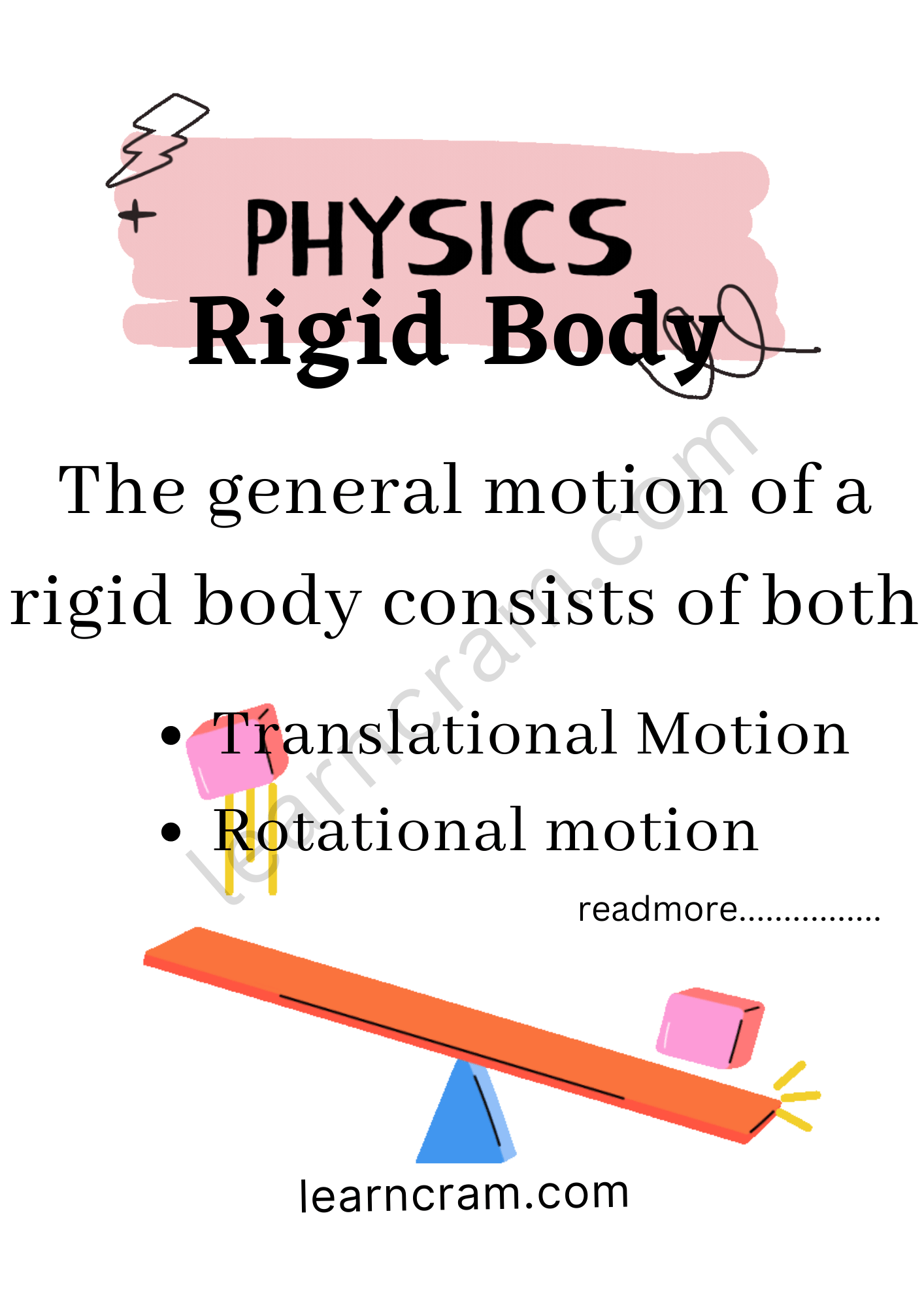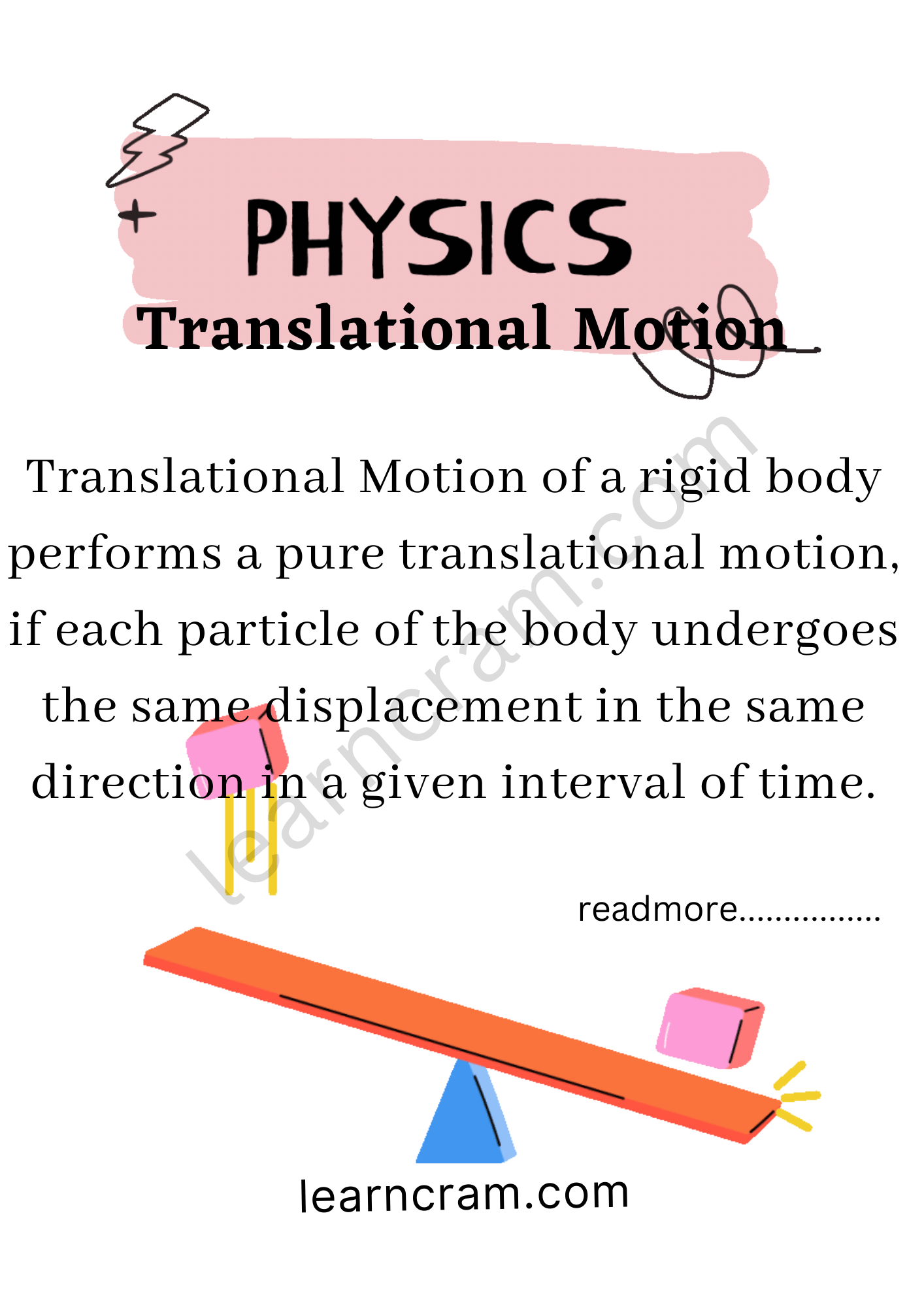# Rigid Body in Physics | Definition, Example, Types – Rotational Motion

Rigid Body Definition:
A body is said to be a rigid body, when it has perfectly definite shape and size. The distance between all points of particles of such a body do not change, while applying any force on it.

We are giving a detailed and clear sheet on all Physics Notes that are very useful to understand the Basic Physics Concepts.

## Rigid Body in Physics | Definition, Example, Types – Rotational Motion

The general motion of a rigid body consists of both

• Translational Motion
• Rotational motionTranslational Motion in Physics:
Translational Motion of a rigid body performs a pure translational motion, if each particle of the body undergoes the same displacement in the same direction in a given interval of time.Rotational Motion Physics:
Rotational Motion of a rigid body performs a pure rotational motion, if each particle of the body moves in a circle, and the centre of all the circles lie on a straight line called the axes of rotation.

Equations of Rotational Motion:
(i) ω = ω0 + αt
(ii) θ = ω0t + $$\frac{1}{2}$$αt²
(iii) ω² = ω²0 + 2αθ

Rigid Body Example in Physics:
A ball bearing made of hardened steel is a good example of a rigid body. Now, drop a ball bearing on a polished marble floor — it’ll bounce just about as well as a Superball. Why’s that? Because, though it is a rigid body, it has near-perfect elasticity.

Rotational Motion:
In this portion, we will learn about the rotational motion of the objects. A body moves completely in rotational motion when each particle of the body moves in a circle about a single line. When a force is applied on a body about an axis it causes a rotational motion. The force applied here is called the torque. The axis of the rotation usually goes through the body. Also, learn the two theorems such as parallel axes and perpendicular theorem explained with respect to rotational motion of objects.